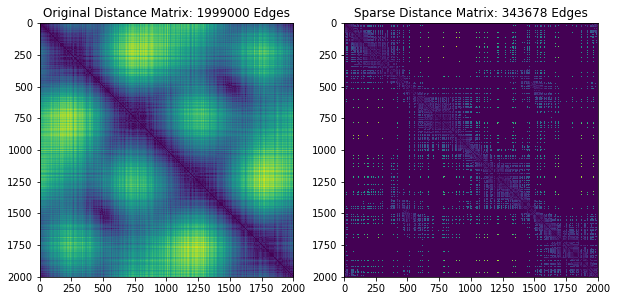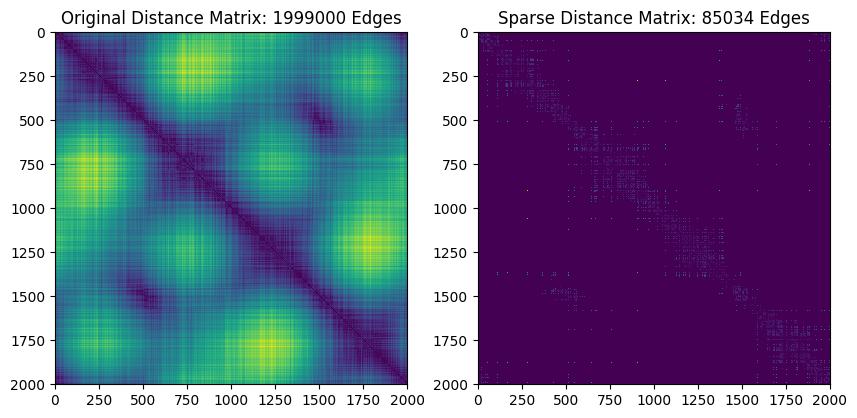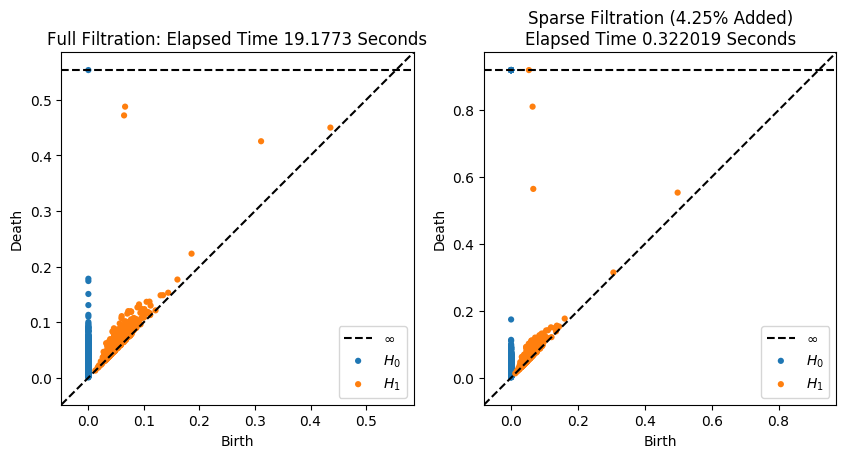# Approximate Sparse Filtrations¶

In this module, we will explore an approximation algorithm which is meant to reduce the run time of the persistence algorithm . For more information on this algorithm, please view the following video:

 Cavanna, Nicholas J., Mahmoodreza Jahanseir, and Donald R. Sheehy. “A geometric perspective on sparse filtrations.” Proceedings of the Canadian Conference on Computational Geometry (CCCG 2015).

:

from ripser import ripser
from persim import plot_diagrams

import numpy as np
import matplotlib.pyplot as plt
from sklearn.metrics.pairwise import pairwise_distances
from scipy import sparse
import time


Now, we define a “greedy permutation,” as a function which performs furthest points sampling, a key step in the algorithm used to determine “insertion radii” $$\lambda_i$$ for each point. For an animation of how this works, please visit:

https://gist.github.com/ctralie/128cc07da67f1d2e10ea470ee2d23fe8

:

def getGreedyPerm(D):
"""
A Naive O(N^2) algorithm to do furthest points sampling

Parameters
----------
D : ndarray (N, N)
An NxN distance matrix for points

Return
------
lamdas: list
"""

N = D.shape
#By default, takes the first point in the permutation to be the
#first point in the point cloud, but could be random
perm = np.zeros(N, dtype=np.int64)
lambdas = np.zeros(N)
ds = D[0, :]
for i in range(1, N):
idx = np.argmax(ds)
perm[i] = idx
lambdas[i] = ds[idx]
ds = np.minimum(ds, D[idx, :])
return lambdas[perm]


Now, we define a function which, given a distance matrix representing a point cloud, a set of insertion radii, and an approximation factor $$\epsilon$$, returns a sparse distance matrix with re-weighted edges, whose persistence diagrams are each guaranteed to be a $$(1+\epsilon)$$ multiplicative approximation of the true persistence diagrams (see )

:

def getApproxSparseDM(lambdas, eps, D):
"""
Purpose: To return the sparse edge list with the warped distances, sorted by weight

Parameters
----------
lambdas: list
eps: float
epsilon approximation constant
D: ndarray
NxN distance matrix, okay to modify because last time it's used

Return
------
DSparse: scipy.sparse
A sparse NxN matrix with the reweighted edges
"""
N = D.shape
E0 = (1+eps)/eps
E1 = (1+eps)**2/eps

# Create initial sparse list candidates (Lemma 6)
# Search neighborhoods
nBounds = ((eps**2+3*eps+2)/eps)*lambdas

# Set all distances outside of search neighborhood to infinity
D[D > nBounds[:, None]] = np.inf
[I, J] = np.meshgrid(np.arange(N), np.arange(N))
idx = I < J
I = I[(D < np.inf)*(idx == 1)]
J = J[(D < np.inf)*(idx == 1)]
D = D[(D < np.inf)*(idx == 1)]

#Prune sparse list and update warped edge lengths (Algorithm 3 pg. 14)
minlam = np.minimum(lambdas[I], lambdas[J])
maxlam = np.maximum(lambdas[I], lambdas[J])

# Rule out edges between vertices whose balls stop growing before they touch
# or where one of them would have been deleted.  M stores which of these
# happens first
M = np.minimum((E0 + E1)*minlam, E0*(minlam + maxlam))

t = np.arange(len(I))
t = t[D <= M]
(I, J, D) = (I[t], J[t], D[t])
minlam = minlam[t]
maxlam = maxlam[t]

# Now figure out the metric of the edges that are actually added
t = np.ones(len(I))

# If cones haven't turned into cylinders, metric is unchanged
t[D <= 2*minlam*E0] = 0

# Otherwise, if they meet before the M condition above, the metric is warped
D[t == 1] = 2.0*(D[t == 1] - minlam[t == 1]*E0) # Multiply by 2 convention
return sparse.coo_matrix((D, (I, J)), shape=(N, N)).tocsr()


Now let’s set up a point cloud we can test this on, which has enough points for ripser to start slowing down a bit. We’ll perform the full rips filtration on this point cloud as a ground truth, and we will time it

:

X = tadasets.infty_sign(n=2000, noise=0.1)
plt.scatter(X[:, 0], X[:, 1])

tic = time.time()
resultfull = ripser(X)
toc = time.time()
timefull = toc-tic
print("Elapsed Time: %.3g seconds, %i Edges added"%(timefull, resultfull['num_edges']))

Elapsed Time: 19.2 seconds, 1999000 Edges addedNow let’s run an approximate version and plot $$H_1$$ for both next to each other

:

eps = 0.1

# Compute the sparse filtration
tic = time.time()

# First compute all pairwise distances and do furthest point sampling
D = pairwise_distances(X, metric='euclidean')
lambdas = getGreedyPerm(D)

# Now compute the sparse distance matrix
DSparse = getApproxSparseDM(lambdas, eps, D)

# Finally, compute the filtration
resultsparse = ripser(DSparse, distance_matrix=True)
toc = time.time()
timesparse = toc-tic
print("Elapsed Time: %.3g seconds, %i Edges added"%(timesparse, resultsparse['num_edges']))

Elapsed Time: 1.24 seconds, 343678 Edges added

:

# Plot the sparse distance matrix and edges that were added
plt.figure(figsize=(10, 5))
plt.subplot(121)
D = pairwise_distances(X, metric='euclidean')
plt.imshow(D)
plt.title("Original Distance Matrix: %i Edges"%resultfull['num_edges'])
plt.subplot(122)
DSparse = DSparse.toarray()
DSparse = DSparse + DSparse.T
plt.imshow(DSparse)
plt.title("Sparse Distance Matrix: %i Edges"%resultsparse['num_edges'])

# And plot the persistence diagrams on top of each other
plt.figure(figsize=(10, 5))
plt.subplot(121)
plot_diagrams(resultfull['dgms'], show=False)
plt.title("Full Filtration: Elapsed Time %g Seconds"%timefull)
plt.subplot(122)
plot_diagrams(resultsparse['dgms'], show=False)Now we’ll do the exact same thing, but this time we’ll raise epsilon to get a faster, slightly worse approximation

:

eps = 0.4

# Compute the sparse filtration
tic = time.time()

# First compute all pairwise distances and do furthest point sampling
D = pairwise_distances(X, metric='euclidean')
lambdas = getGreedyPerm(D)

# Now compute the sparse distance matrix
DSparse = getApproxSparseDM(lambdas, eps, D)

# Finally, compute the filtration
resultsparse = ripser(DSparse, distance_matrix=True)
toc = time.time()
timesparse = toc-tic
print("Elapsed Time: %.3g seconds, %i Edges added"%(timesparse, resultsparse['num_edges']))

Elapsed Time: 0.322 seconds, 85034 Edges added

:

#Plot the sparse distance matrix and edges that were added
plt.figure(figsize=(10, 5))
plt.subplot(121)
D = pairwise_distances(X, metric='euclidean')
plt.imshow(D)
plt.title("Original Distance Matrix: %i Edges"%resultfull['num_edges'])
plt.subplot(122)
DSparse = DSparse.toarray()
DSparse = DSparse + DSparse.T
plt.imshow(DSparse)
plt.title("Sparse Distance Matrix: %i Edges"%resultsparse['num_edges'])

#And plot the persistence diagrams on top of each other
plt.figure(figsize=(10, 5))
plt.subplot(121)
plot_diagrams(resultfull['dgms'], show=False)
plt.title("Full Filtration: Elapsed Time %g Seconds"%timefull)
plt.subplot(122)Not bad for nearly two orders of magnitude faster! Mainly, the death times just got a little bit later for the two most important classes in $$H_1$$
[ ]: